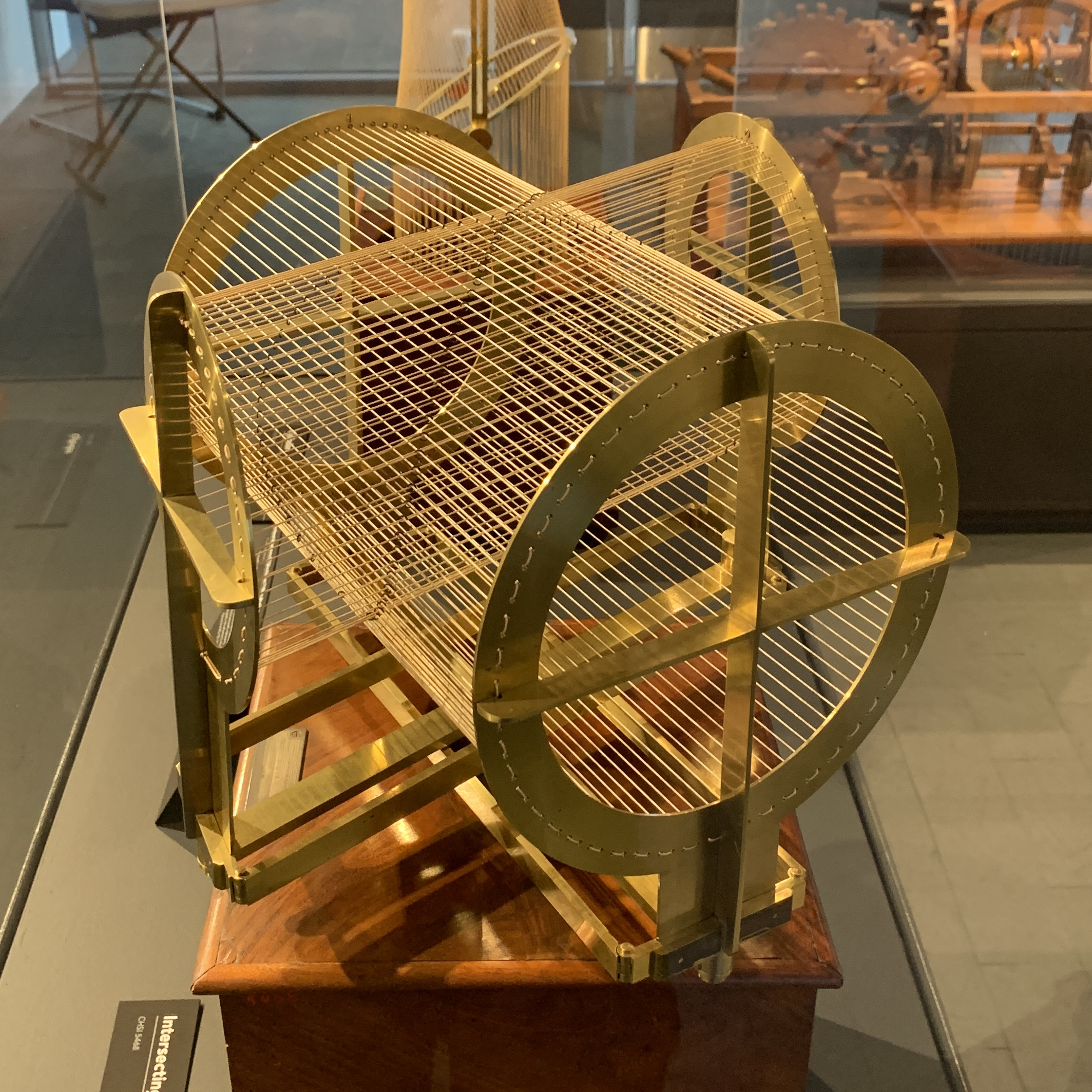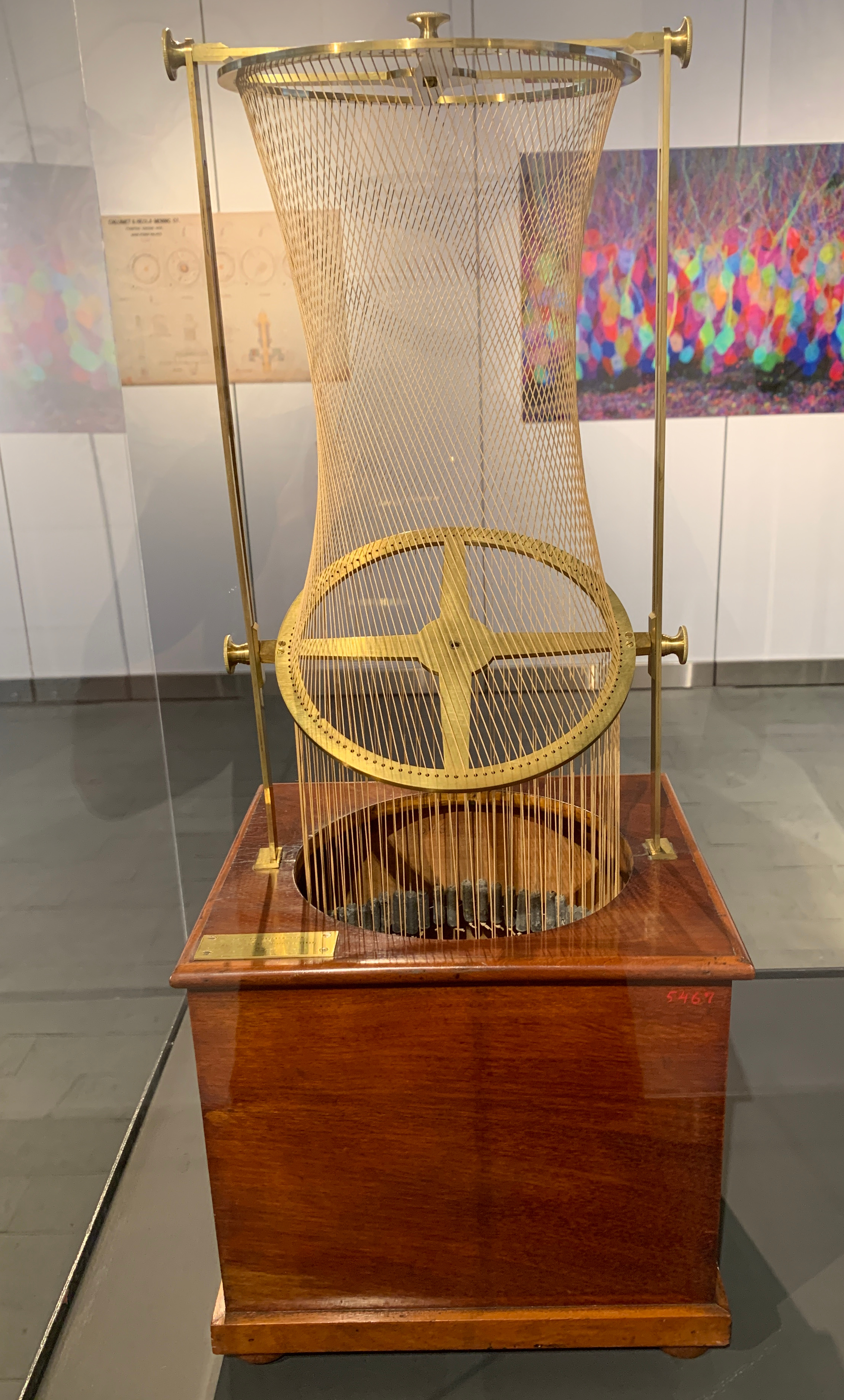# Intersecting cylinders

The special exhibit Visual Science in the Harvard Science center (2nd floor) shows a nice model of two intersecting cylinders. The intersection of two cylinders of the same radius has already been studied by Archimedes. When taking two arbitrary cylinders, the intersection curve can become much more interesting. In this example, one sees a variant of the Viviani curve, an algebraic curve which has the shape of a figure 8.Also a nice figure of a hyperboloid: